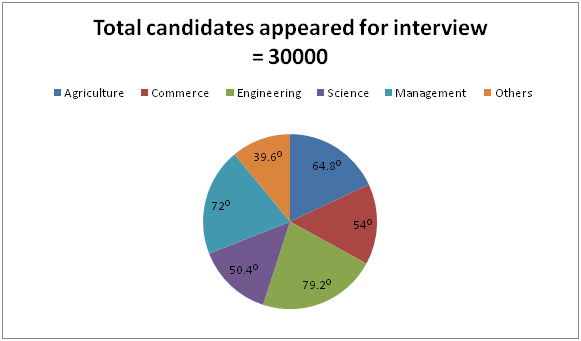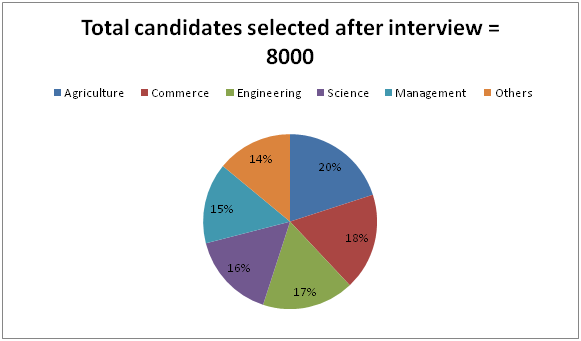# IBPS RRB PO Mains Quantitative Aptitude Questions 2019 (Day-06)

Dear Aspirants, Our IBPS Guide team is providing new series of Quantitative Aptitude Questions for IBPS RRB PO Mains 2019 so the aspirants can practice it on a daily basis. These questions are framed by our skilled experts after understanding your needs thoroughly. Aspirants can practice these new series questions daily to familiarize with the exact exam pattern and make your preparation effective.

Check here for IBPS RRB PO Mains Mock Test 2019

Check here for IBPS RRB Clerk Mains Mock Test 2019

Check here for IBPS PO Prelims Mock Test 2019

### Click Here to Subscribe Crack High Level Puzzles & Seating Arrangement Questions PDF 2019 Plan

[WpProQuiz 6904]

Directions (1 – 5): Study the following information carefully and answer the given questions.

The following pie chart1 shows the percentage distribution of total number of candidates appeared for the interview from various disciplines from a certain city.The pie chart2 shows the percentage distribution of total number of candidates selected after the interview from those disciplines.1) Find the difference between the total number of candidates appeared for the interview from Commerce and Engineering discipline to that of total number of candidates selected after the interview from Agriculture and Management discipline.

a) 9200

b) 9800

c) 8300

d) 8700

e) None of these

2) Total number of candidates appeared for the interview from Agriculture and Science discipline together is approximately what percentage of total number of candidates selected after the interview from Engineering and Other discipline together?

a) 456 %

b) 387 %

c) 312 %

d) 479 %

e) 273 %

3) Find the ratio between the total number of candidates appeared for the interview from Management discipline to that of total number of candidates selected after the interview from Commerce discipline.

a) 17: 11

b) 33: 19

c) 49: 33

d) 25: 6

e) None of these

4) Find the difference between the average number of candidates appeared for the interview from Science and Other discipline together to that of the average number of candidates selected after the interview from Agriculture and Science discipline together.

a) 2310

b) 2150

c) 2470

d) 2530

e) None of these

5) Total number of candidates appeared for the interview from Commerce and Management discipline together is approximately what percentage more than the total number of candidates selected after interview from Engineering and Science discipline together?

a) 75 % less

b) 90 % more

c) 75 % more

d) 90 % less

e) 115 % more

Directions (6 – 10): Find out the wrong number in the following number series.

6) 13, 77, 202, 418, 763, 1273

a) 418

b) 763

c) 1273

d) 202

e) 77

7) 53760, 6720, 960, 160, 80, 8

a) 8

b) 80

c) 960

d) 6720

e) 160

8) 80, 120, 300, 1040, 4725, 25987.5

a) 120

b) 1040

c) 4725

d) 25987.5

e) 300

9) 325, 362, 401, 442, 484, 530

a) 530

b) 484

c) 442

d) 401

e) 362

10) 19, 20, 45, 129, 520, 2605

a) 45

b) 129

c) 520

d) 2605

e) 20

Direction (1-5) :

The total number of candidates appeared for the interview from Commerce and Engineering discipline

= >30000 * [(54 + 79.2)/360] = 11100

Total number of candidates selected after the interview from Agriculture and Management discipline

= >8000 * [(20 + 15)/100] = 2800

Required difference = 11100 – 2800 = 8300

Total number of candidates appeared for the interview from Agriculture and Science discipline together

= >30000 * [(64.8 + 50.4)/360] = 9600

Total number of candidates selected after the interview from Engineering and Other discipline together

= >8000 * [(17 + 14)/100] = 2480

Required % = (9600/2480) * 100 = 387.096 % = 387 %

The total number of candidates appeared for the interview from Management discipline

= >30000 * (72/360)

The total number of candidates selected after the interview from Commerce discipline

= >8000 * (18/100)

Required ratio = [30000 * (72/360)]: [8000 * (18/100]

= 25: 6

The average number of candidates appeared for the interview from Science and Other discipline together

= >30000 * [(50.4 + 39.6)/360] * (1/2) = 3750

The average number of candidates selected after the interview from Agriculture and Science discipline together

= >8000 * [(20 + 16)/100] * (1/2) = 1440

Required difference = 3750 – 1440 = 2310

Total number of candidates appeared for the interview from Commerce and Management discipline together

= > 30000 * [(54 + 72)/360] = 10500

Total number of candidates selected after interview from Engineering and Science discipline together

= >8000 * [(17 + 16)/100] = 2640

Required % = [(10500 – 2640)/10500] * 100 = 74.857 % = 75 % more

Direction (6-10) :

13 + 43 = 77

77 + 53 = 202

202 + 63 = 418

418 + 73 = 761

761 + 83 = 1273

The wrong term is 763

53760 ÷ 8 = 6720

6720 ÷ 7 = 960

960 ÷ 6 = 160

160 ÷ 5 = 32

32 ÷ 4 = 8

The wrong term is 80

80 * 1.5 = 120

120 * 2.5 = 300

300 * 3.5 = 1050

1050 * 4.5 = 4725

4725 * 5.5 = 25987.5

The wrong term is 1040

182 + 1 = 325

192 + 1 = 362

202 + 1 = 401

212 + 1 = 442

222 + 1 = 485

232 + 1 = 530

The wrong term is 484

19 * 1 + 1 = 20

20 * 2 + 2 = 42

42 * 3 + 3 = 129

129 * 4 + 4 = 520

520 * 5 + 5 = 2605

The wrong term is 45